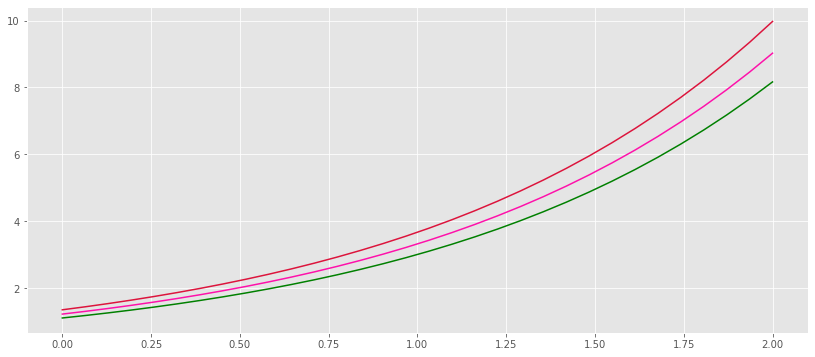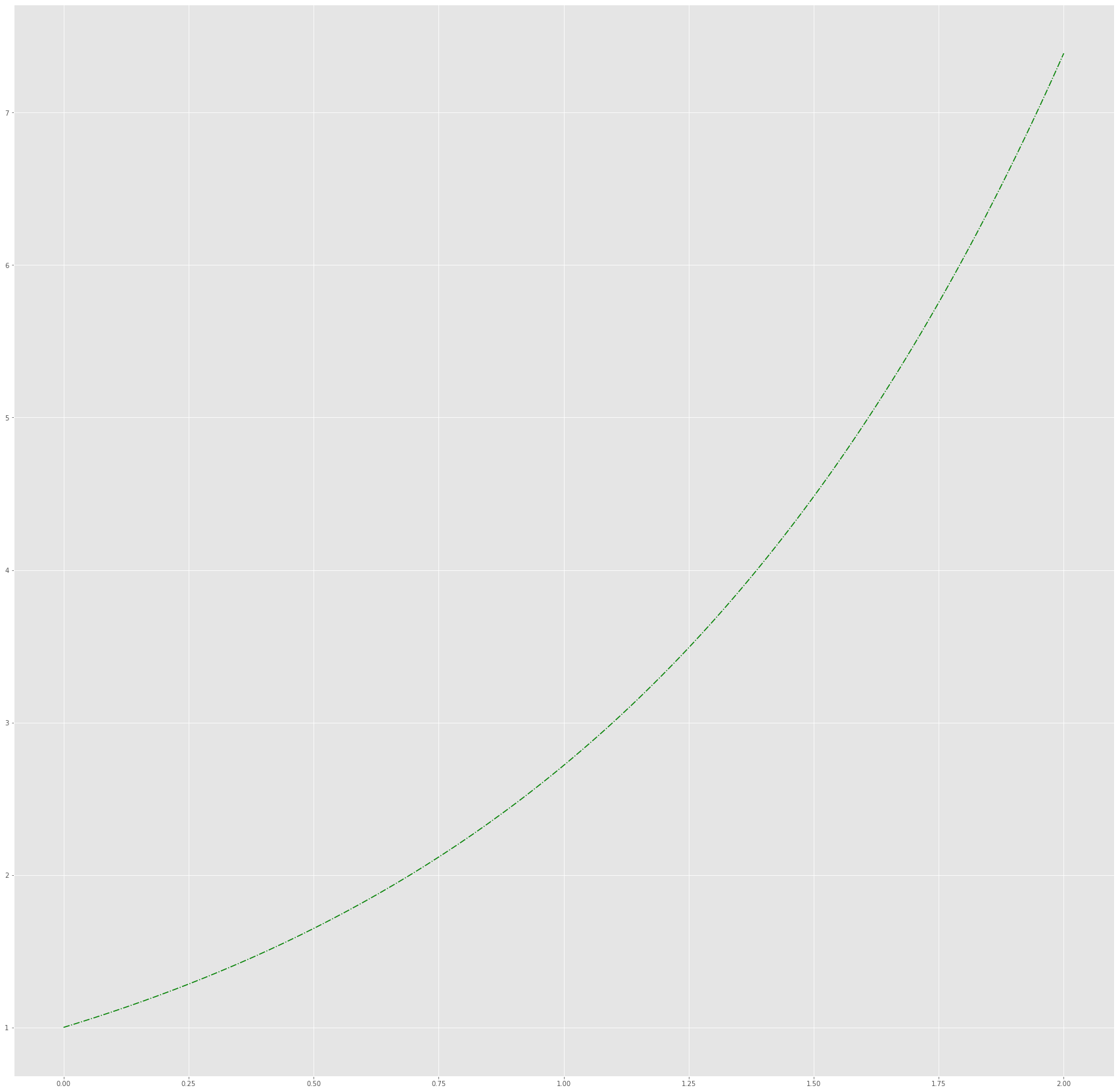03.05 Plotting Engines¶

We now know a good deal about making figures with matpotlib but there's, of course, much, much more. Two things that we did not yet touch are matplotlib interfaces outside of jupyter, and its interfaces to other libraries.plt-engine.svg

Let's import matplotlib the normal jupyter way for now.

In :
import numpy as np
import matplotlib.pyplot as plt
%matplotlib inline

Styles¶

One can use style-sheets with matplotlib, these style-sheets contain default configuration that alter the look and feel of all plots. Many higher level interfaces use style-sheets to integrate into matplotlib, to list the installed style-sheets we can perform the following.

The styles with talk and poster in their names increase the size of all labels. We will use some of these styles from here on in order to read graphs easier.

In :
plt.style.available
Out:
['seaborn-bright',
'fast',
'ggplot',
'seaborn-dark',
'seaborn-muted',
'seaborn-ticks',
'grayscale',
'seaborn-notebook',
'_classic_test',
'seaborn-white',
'classic',
'seaborn-pastel',
'seaborn-whitegrid',
'seaborn-talk',
'Solarize_Light2',
'seaborn',
'seaborn-dark-palette',
'seaborn-deep',
'seaborn-paper',
'tableau-colorblind10',
'dark_background',
'seaborn-poster',
'seaborn-colorblind',
'bmh',
'seaborn-darkgrid',
'fivethirtyeight']

And to enable one such style one would.

In :
plt.style.use('ggplot')

Unfortunately applying a second style (i.e. changing styles) on the fly does not really work. The style-sheet changes matplotlib globals, which another style may not adapt.

That said, one can use plt.style.context to enable a style for a small part of code. Or create one's own styles with style sheets.

For now let's have a look at the style we applied:

In :
fig, ax = plt.subplots(figsize=(14, 6))
x = np.linspace(0, 2, 32)

ax.plot(x, np.exp(x + 0.1), color='green')
ax.plot(x, np.exp(x + 0.2), color='#fe11aa')
ax.plot(x, np.exp(x + 0.3), color='crimson');This is quite different from the graphs we saw until now. One can experiment with styles before attempting to customize a graph to their liking.

show()?¶

In matplotlib code outside of jupyter the show function is almost always used. The show functions tells the matplotlib backed to actually draw and display the figure. Inside jupyter the %matplotlib inline magic does that for us automatically. (In reality %matplotlib inline saves the figure to memory, computes the base64 representation of it and injects a data: URL into the notebook). Within IPython the %matplotlib magic functions similarly, it will generate, and update, an image everytime a plotting function is called.

Yet, when we are not working interactively (Jupyter, IPython, or even something else) we do not always want to open a window with an image. For example, script that should generate graphs on disk on headless servers probably have no need to display the images (and that would fail anyway). The show function in scripts is an explicit way of telling matplotlib to actually draw and show the image.

Yet note that show can only be called once in a script. It sets several global values in the backend display engine, and these values may not be optimal for all plots. (i.e. a second call to show may result in an ugly plot, or even a plain error.)

For example to make a script showing an image one would do:

import numpy as np
import matplotlib.pyplot as plt

x = np.linspace(0, 2, 128)

fig, ax = plt.subplots(figsize=(30, 30))
ax.plot(x, np.exp(x), '-.g')

plt.show()

Save the Graph¶

If we do not want to show a graph we probably want to save it to a file - or send it over the network, or similar. The previous script can save the figure instead of displaying it:

import numpy as np
import matplotlib.pyplot as plt

x = np.linspace(0, 2, 128)

fig, ax = plt.subplots(figsize=(30, 30))
ax.plot(x, np.exp(x), '-.g')

fig.savefig('natural_exponent.png', dpi=300)

But we can save graphs from Jupyter too. We simply use savefig on the figure object. We saw that the default DPI (dots per inch) in Jupyter is around 72 DPI, that is good enough for display but certainly not good enough for printing. savefig= accepts a dpi= parameter to change the DPI to a value appropriate for the consuming application of the image.

Note that the %matplotlib inline magic will still display the image.

In :
fig, ax = plt.subplots(figsize=(30, 30))
x = np.linspace(0, 2, 128)
ax.plot(x, np.exp(x), '-.g')
fig.savefig('natural_exponent.png', dpi=300);PNG (Portable Network Graphics) is the default format in matplotlib but, by far, not the only one. Let's get the list of supported formats in our installation.

In :
fig.canvas.get_supported_filetypes()
Out:
{'ps': 'Postscript',
'eps': 'Encapsulated Postscript',
'pdf': 'Portable Document Format',
'pgf': 'PGF code for LaTeX',
'png': 'Portable Network Graphics',
'raw': 'Raw RGBA bitmap',
'rgba': 'Raw RGBA bitmap',
'svg': 'Scalable Vector Graphics',
'svgz': 'Scalable Vector Graphics',
'jpg': 'Joint Photographic Experts Group',
'jpeg': 'Joint Photographic Experts Group',
'tif': 'Tagged Image File Format',
'tiff': 'Tagged Image File Format'}

Extra: current Working Directory in Jupyter¶

OK, we apparently saved an image to disk but where it is?

In Python all paths are understood in the UNIX fashion:

• Paths starting with / are absolute paths
• All other paths are relative to the current working directory

Jupyter sets the current working directory of its kernel - the engine actually executing the code - to the path of the notebook itself. Therefore our image is in the same directory as the notebook we are running.

Note: In Python you can (and should) use / as the path separator on both UNIX-like and MS Windows systems.

Other engines¶

matplotlib is less clunky in recent versions than it was a handful of years ago. But the clunkiness is of the interface into it and its plotting defaults. The plotting engine of matplotlib is sturdy and deals well with many visualization problems. Despite that, over the years other plotting interfaces have been developed, some of which extend matplotlib with more plot types, some change the appearance of plots. Some very well known extensions include:

• ggplot simulates plotting from the R environment. For a long time its graphs had better appearance than matplotlib defaults. It also works better with pandas than plain matplotlib does. That said, behind the scened ggplot calls the matplotlib engine for the plotting.

• seaborn extends matplotlib with several statistics oriented graphs. All seaborn does is to utilize the matplotlib engine to draw more complex graphs. That said, if one needs specific graphs: voilinplots, boxplots, heatmaps, joint distribution plots or cluster maps; one can just use the seaborn interfaces instead of writing such a plot from scratch in matplotlib.

Once the amount of data surpasses the memory of the machine we are working with, or we need interactive plots, matplotlib is placed out of its depth. For such problem completely different plotting engines are needed. Some such engines are called together the pyViz group, for Python Visualization. Some libraries there are:

• bokeh provides a way of generating, from Python, web pages that will display data and allow for interactive graphs. The data can be provided in chunks, allowing for big amounts of data to be used for the plotting.

• holoviews uses bokeh, or possibly another renderer, to plot visualizations. Where visualizations are plots or other aspects of the data which can be useful during data analysis.

• dask is a cluster engine to distribute the plotting across several machines. When one needs to plot more data that would fit in the memory of any machine, dask is the tool in Python for the job.

• d3js is a JavaScript library but it is often used in combination with Python webservers to create visualizations.

• pyecharts is another library focused on JavaScript animations generated from Python code. It can modify its JavaScript on the fly, and has good understanding of browser behaviour. Hence it can be used as a substitute for matplotlib animations, even inside Jupyter.

matplotlibitself has visualization capabilities under the magic %matplotlib notebook (instead of %matplotlib inline) but these are quite limited.